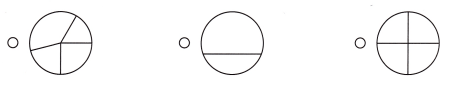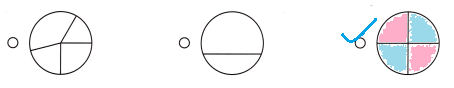# Texas Go Math Grade 2 Lesson 4.1 Answer Key Equal Parts

Refer to our Texas Go Math Grade 2 Answer Key Pdf to score good marks in the exams. Test yourself by practicing the problems from Texas Go Math Grade 2 Lesson 4.1 Answer Key Equal Parts.

## Texas Go Math Grade 2 Lesson 4.1 Answer Key Equal Parts

Explore

Put pattern blocks together to match the shape of the hexagon. Trace the shape you made.

FOR THE TEACHER • Have children place a yellow hexagon pattern block on the workspace and make the same shape by using any combination of pattern blocks. Discuss how they know if the outline of the blocks they used is the same shape as the yellow hexagon.
Yes, children make the same shape by using any combination of pattern blocks.Explanation:
A yellow hexagon pattern block on the workspace and make the same shape by using any combination of 6 triangle pattern blocks are joined to form a same yellow hexagon

Math Talk
Mathematical Processes

Describe how the shapes you used are different from the shapes a classmate used.
Shapes are described depending on the number of its sides and to some degree its angular relationship. Shapes of classmate are described by virtue of the area of space bordered by the lines.

Model and Draw

The green rectangle is the whole. It can be divided into equal parts.Share and Show

Write how many equal parts there are in the whole. Write halves, fourths, or eighths to name the equal parts.

Question 1.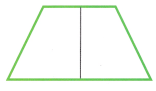___________ equal parts
2 halves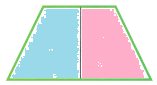two equal parts
Explanation:
On dividing the whole or a object into equal parts, we get 2 halves.
We need to divide an object or a whole number into equal parts to distribute it equally.
These equal parts have to be the same in measurements like weight, volume, dimensions, numbers etc.

Question 2.___________ equal parts
8 equal partsExplanation:
A whole can be divided into parts of equal size.
The above rectangle has been divided into eight equal parts.

Question 3.___________ equal parts
4 equal parts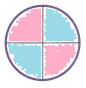Explanation:
A whole can be divided into parts of equal size.
The above circle has been divided into 4 equal parts.

Question 4.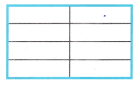___________ equal parts
8 equal parts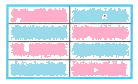Explanation:
A whole can be divided into parts of equal size.
The above rectangle has been divided into eight equal parts.

Question 5.___________ equal parts
2 equal parts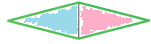Explanation:
On dividing the whole or a object into equal parts, we get 2 halves.
We need to divide an object or a whole number into equal parts to distribute it equally.
These equal parts have to be the same in measurements like weight, volume, dimensions, numbers etc.

Question 6.___________ equal parts
4 equal partsExplanation:
A whole can be divided into parts of equal size.
The above rectangle has been divided into 4 equal parts.

Problem Solving

Write how many equal parts there are in the whole. Write halves, fourths, or eighths to name the equal parts.

Question 7.___________ equal parts
2 equal partsExplanation:
On dividing the whole or a object into equal parts, we get 2 halves.
We need to divide an object or a whole number into equal parts to distribute it equally.
These equal parts have to be the same in measurements like weight, volume, dimensions, numbers etc.

Question 8.___________ equal parts
4 equal partsExplanation:
A whole can be divided into parts of equal size.
The above square has been divided into 4 equal parts.

Question 9.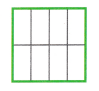___________ equal parts
8 equal partsExplanation:
A whole can be divided into parts of equal size.
The above square has been divided into eight equal parts.

Question 10.
H.O.T. Draw to show halves. Explain how you know that the parts are halves.The complete picture or object is divided in to two equal parts is called halves as shown in below pictures.Explanation:
On dividing the whole or a object into equal parts, we get 2 halves.
We need to divide an object or a whole number into equal parts to distribute it equally.
These equal parts have to be the same in measurements like weight, volume, dimensions, numbers etc.

Question 11.
H.O.T. Multi-Step Sort the shapes.

• Use red to color the shapes that show eighths.
• Use blue to color the shapes that show fourths.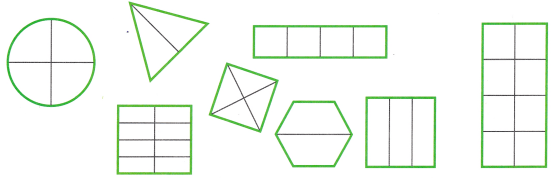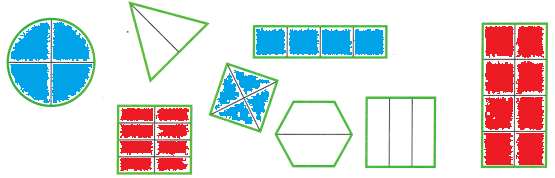Explanation:
As, we know from the above exercises about halves, fourths and eighths in detail.
So, from the above pictures circle, rectangle and square are divided into fourths colored with blue and eighths colored with red.

Question 12.
Analyze Joe drew a circle divided into fourths. Which shape has parts that are fourths?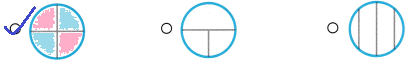Explanation:
Option (A) shows fourths.
If a whole is divided into four equal parts, each part is a FOURTH.
A fourth is obtained by dividing a whole (1) by 4.

Question 13.
Use Math Language Anna drew a rectangle divided into eighths. Which shape has parts that are eighths?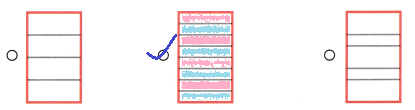Explanation:
Option (B) shows eights.
If a whole is divided into eight equal parts, each part is an Eighth.
An eighth is obtained by dividing a whole (1) by 8.

Question 14.
TEXAS Test Prep Which shape has parts that are fourths?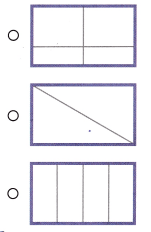Explanation:
Option (C) shows fourths.
If a whole is divided into four equal parts, each part is a FOURTH.
A fourth is obtained by dividing a whole (1) by 4.

TAKE HOME ACTIVITY • Ask your child to fold one sheet of paper into halves and another sheet of paper into fourths.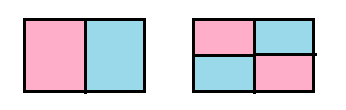Explanation:
When the child folds the paper sheet, he will get the above images.
If a whole is divided into two equal parts, each part is a Halves.
A halves is obtained by dividing a whole (1) by 2.
If a whole is divided into four equal parts, each part is a FOURTH.
A fourth is obtained by dividing a whole (1) by 4.

### Texas Go Math Grade 2 Lesson 4.1 Homework and Practice Answer Key

Write how many equal parts there are in the whole. Write halves, fourths, or eighths to name the equal parts.

Question 1.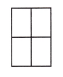Fourths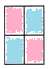Explanation:
The above picture is of fourths, it has four equal parts.
A fourth is obtained by dividing a whole (1) by 4.

Question 2.HalvesExplanation:
The above picture is of halves, it has two equal parts.
A half is obtained by dividing a whole (1) by 2.

Question 3.Eighths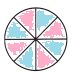Explanation:
The above picture is of eighths, it has eight equal parts.
A eighth is obtained by dividing a whole (1) by 8.

Problem Solving

Draw to solve.

Question 4.
Multi-Step Sort the shapes.

• Use red to color the shapes that show eighths.
• Use blue to color the shapes that show fourths.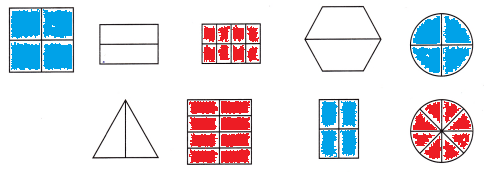Explanation:
If a whole is divided into 8 equal parts, each part is an eighth.
An eighth is obtained by dividing a whole (1) by 8.
So, color all the eighths with red after identifying.
If a whole is divided into four equal parts, each part is a FOURTH.
A fourth is obtained by dividing a whole (1) by 4.
So, color all the fourths with blue after identifying.

Lesson Check

Question 5.
Which shape has parts that show fourths?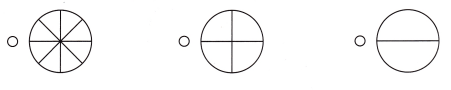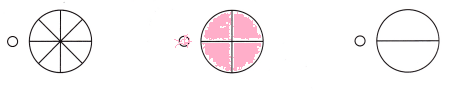Explanation:
Option (B) shows the fourths,
If a whole is divided into four equal parts, each part is a FOURTH.
A fourth is obtained by dividing a whole (1) by 4.

Question 6.
Which shape has parts that show halves?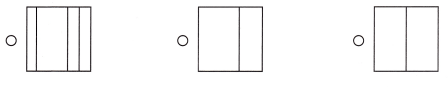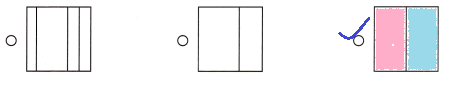Explanation:
From the above pictures option (C) shows halves.
If a whole is divided into 2 equal parts, each part is a Half.
A Half is obtained by dividing a whole (1) by 2.

Question 7.
Which shape has parts that show eighths?Explanation:
In the above pictures option (A) shows eighths.
If a whole is divided into 8 equal parts, each part is a Eighth.
A Eighth is obtained by dividing a whole (1) by 8.

Question 8.
The cheese pizza was divided into fourths. Which pizza is divided into fourths?